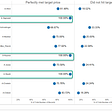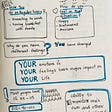# Linear Regression & Gradient Descent : Described Simply without Scikit Library Implementation

`def mean(arr):sum = 0for i in range(0, len(arr)):sum = sum + arr[i]return sum / len(arr)def covariance(arr1, arr2):sum = 0for i in range(0, len(arr1)):sum = (sum + (arr1[i] - mean(arr1)) *(arr2[i] - mean(arr2)))return sum / (len(arr1) - 1)def variance(x):xbar = mean(x)mean_difference_squared_readings = [pow((x - xbar), 2) for xs in x]variance = sum(mean_difference_squared_readings)return variance/float(len(x)-1)def beta(x,y):beta = covariance(x,y) / variance(x)return betadef epsilon(x, y):epsilon = mean(y ) - (beta(x,y)*mean(x))return epsilon`
`a = epsilon(df1,df2)b = beta(df1,df2)print(a,b)`
`array([ -791.5065989 , -1309.24081572, -1700.44334054, ...,         -278.33176374,   181.59598996, -2604.84740872])array([0.50833517, 0.70749599, 0.85798289, ..., 0.31092824, 0.13400428,        1.20588698])`
`sns.lineplot(x=b, y=a)`
`x_nought = 3 #wheer the algorithm startsalpha = 0.01 #alpha_learning_rateprecision = 0.000001previous_step_size  = 1max_iters = 10000 #maximum numbers of iterations that need to be carriediters =0 #initiating the iter counterdf = lambda x: 2*(x+10) #gradient of our functionplot = []iter_plot = []while previous_step_size > precision and iters < max_iters:prev_x = x_nought #store current x value in prev_xx_nought = x_nought - alpha*df(prev_x) #grad descentprevious_step_size = abs(x_nought - prev_x)# change in xiters = iters+1 #iteration countprint('Iterations', iters, 'X value is', x_nought) #prints all the iterationsprint('The local minima is', x_nought,'Occurs at',iters,'th iteration')`
`Iterations 1 X value is 2.74 Iterations 2 X value is 2.4852000000000003 Iterations 3 X value is 2.2354960000000004 Iterations 4 X value is 1.9907860800000003 Iterations 5 X value is 1.7509703584000003..Iterations 616 X value is -9.99994880754244 Iterations 617 X value is -9.999949831391591 Iterations 618 X value is -9.999950834763759 Iterations 619 X value is -9.999951818068483The local minima is -9.999951818068483 Occurs at 619 th iteration`
`sns.lineplot(x=plot, y=iter_plot)`
`sns.distplot(x=plot)`

--

--

--

## More from Akshar Rastogi

Love podcasts or audiobooks? Learn on the go with our new app.

## 3 Algorithms had 100% accuracy this week## Why the C-Suite Needs to be Data Aware## City of Sydney Open Data Sets## Data Science vs Business Intelligence: What’s the Difference?## Visual guide to understanding t-SNE parameters— what they mean.## Sketchnotes- Emotional Intelligence## Personalize the Experience## More from Medium